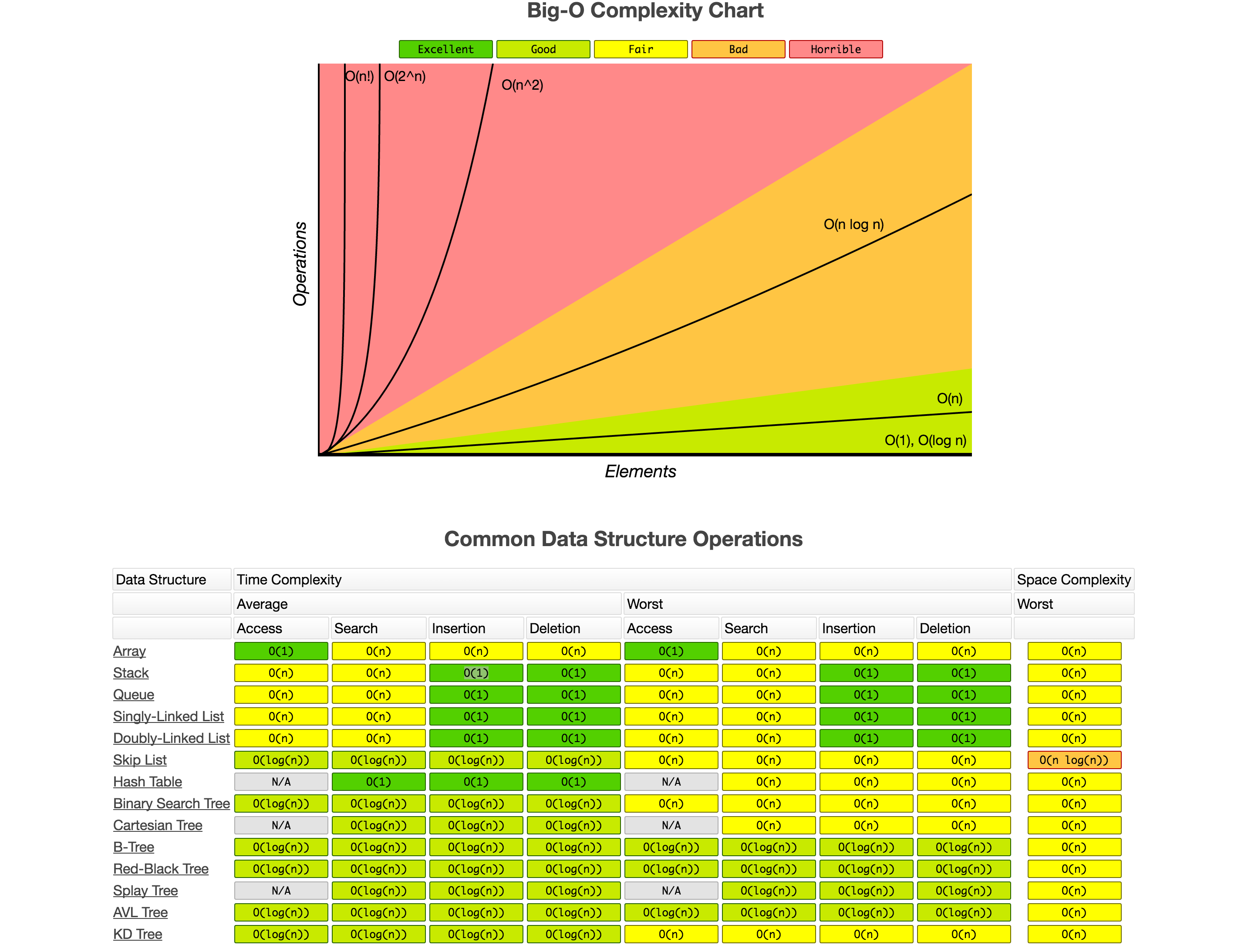# 时间复杂度

$\mathcal{O}$ 记号是算法的上界，我们通常采用的是渐进的分析方法（Asymptotic analysis），即存在足够大的 $n$， 对于规模为 $n$ 的输入，算法需要的基本操作次数为 $\mathcal{O}(n)$。

$\mathcal{O}(1)$ , $\mathcal{O}(\log(n))$ , $\mathcal{O}(n^c)$ 。这样的时间复杂度的问题，我们认为是容易解的问题。

$\mathcal{O}(a^n)$, 例如 $\mathcal{O}(2^n)$ ，是难解的问题，这类算法的计算成本增长极快，通常认为是不可以忍受的。